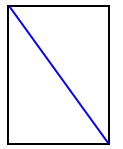English-Vietnamese Dictionary
 ▪ diagnosticate ▪ diagnostician ▪ diagonal ▪ diagonal ▪ diagonal matrix
◊ DIAGONAL
◊diagonal /dai'ægзnl/
▫ tính từ
▪ chéo (đường)
◦ diagonal cloth vải chéo go
▫ danh từ
▪ đường chéo
▪ vải chéo godiagnosticate diagnostician diagonal diagonal diagonal matrix
English Dictionary
 ▪ diagnostician ▪ diagnostics ▪ diagonal ▪ diagonal matrix ▪ diagonalizable
◊ DIAGONAL
diagonal
adj 1: at an angle; especially connecting two nonadjacent corners
of a plane figure or any two corners of a solid that
are not in the same face; "a diagonal line across the
page"
2: having an oblique or slanted direction [syn: {aslant}, {aslope},
{slanted}, {slanting}, {sloped}, {sloping}]
n 1: a straight line that is not at right angles to a side [syn:
{bias}]
2: a punctuation mark (/) used to separate related items of
information [syn: {solidus}, {slash}, {virgule}, {stroke},
{separatrix}]diagnostician diagnostics diagonal diagonal matrix diagonalizable
English-Vietnamese Mining Dictionary
 ▪ diabase ▪ diagenesis ▪ diagonal ▪ diagonal entry ▪ diagonal slicing
◊ DIAGONAL
◊diagonal
đường chéo, đường xiên, (thuộc) đường chéo, đường xiêndiabase diagenesis diagonal diagonal entry diagonal slicing
French-Vietnamese Dictionary
 ▪ diagnose ▪ diagnostic ▪ diagnostiquer ▪ diagonale ▪ diagramme
◊ DID YOU MEAN DÉCAGONAL?
◊décagonal
▫ tính từ
▪ xem décagonne
 diagnose diagnostic diagnostiquer diagonale diagramme
German-Vietnamese Dictionary
 ▪ diagnostisch ▪ diagnostizieren ▪ diagonal ▪ diagonale ▪ diagonalisieren
◊ DIAGONAL
◊diagonal
▪ {cornerwise} theo đường chéo góc
▪ {diagonal} chéo
▪ {transverse} transversal
 diagnostisch diagnostizieren diagonal diagonale diagonalisieren